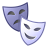# Perpendicular transects at regular intervals

3867
3
02-02-2012 10:03 AMNew Contributor II
I'm working on my thesis research analyzing shoreline change and can't seem to figure out how to cast the transects that I need to. I already have a baseline generated from which I will be able to measure shoreline change. From this baseline I need to cast a perpendicular transect every 75 meters. There are four sites in my research and doing this manually would be too time consuming. Any ideas about how to generate these transects with a python script? I understand that you can write geometries using arcpy, but can't figure out how to generate a line feature with a specified origin, orientation, and length. Any help would be much appreciated.

-Phil
------------------------
Michigan State University
Department of Geography
Tags (3)
3 RepliesbyMVP Honored Contributor
You might be able to adapt this script to your needs. It makes lines perpendicular to roads (culverts) at given points.

```import arcpy, math, random

linefc = arcpy.GetParameterAsText(0) #Roads feature class
pointfc = arcpy.GetParameterAsText(1) #Known stream crossings feature class
linefolder = arcpy.GetParameterAsText(2) #Workspace for culverts output
culvertlen = int(arcpy.GetParameterAsText(3))

#Create intermediate and final feature classes
linefc2 = linefolder + "/culverts" #Culverts file path
pointfc2 = "in_memory/temppoints" #Intermediate, artificial crossings
arcpy.CreateFeatureclass_management("in_memory", "temppoints", "POINT", "#", "#", "#", r"Coordinate Systems/Projected Coordinate Systems/Utm/Nad 1983/NAD 1983 UTM Zone 11N.prj")
arcpy.CreateFeatureclass_management(linefolder, "culverts", "POLYLINE", "#", "#", "#", r"Coordinate Systems/Projected Coordinate Systems/Utm/Nad 1983/NAD 1983 UTM Zone 11N.prj")

#Delete any existing features in culverts or artificial crossings
if arcpy.Exists(pointfc2):
arcpy.DeleteFeatures_management(pointfc2)
if arcpy.Exists(linefc2):
arcpy.DeleteFeatures_management(linefc2)

#Find all crossings within 10m of a road
arcpy.Near_analysis(pointfc, linefc, "10 Meters")

#Find shape field in stream crossing feature class
desc = arcpy.Describe(pointfc)
pointshapefield = desc.ShapeFieldName

#Create search and insert cursors
rows = arcpy.SearchCursor(pointfc)
insrow = arcpy.InsertCursor(pointfc2)

#Enter for loop to create artificial crossings offset within 1m2 from actual crossing points

for row in rows:
#Continue if the crossing is <10m from a road
if row.NEAR_DIST != -1:
feat = row.getValue(pointshapefield)
pnt1 = feat.getPart()
pnt1x = pnt1.X
pnt1y = pnt1.Y
#Offset artificial crossing <=1m in X, <=1m in Y
randomX = random.random()
if randomX%2==0:
randomX = randomX * -1
randomY = random.random()
if randomY%2==0:
randomY = randomY * -1
pnt2x = pnt1.X + randomX
pnt2y = pnt1.Y + randomY
#Insert new point into feature class
feat2 = insrow.newRow()
pnt2 = arcpy.CreateObject("Point")
pnt2.X = pnt2x
pnt2.Y = pnt2y
feat2.shape = pnt2
insrow.insertRow(feat2)

del row
del insrow
del rows

arcpy.Near_analysis(pointfc2, linefc, "11 Meters", "#", "ANGLE")

#Create search and insert cursors
rows2 = arcpy.SearchCursor(pointfc2)
insrow2 = arcpy.InsertCursor(linefc2)

#Find shape field
desc2 = arcpy.Describe(pointfc2)

#Create array to hold new lines
lineArray = arcpy.CreateObject("Array")

#Enter for loop to create culverts

for row2 in rows2:
feat = row2.getValue(desc2.ShapeFieldName)
pnt1 = feat.getPart()
#Create point at far end of culvert
pnt2 = arcpy.CreateObject("Point")
pnt2.X = (math.cos(pnt1rad) * ((culvertlen/2) + row2.NEAR_DIST)) + pnt1.X
pnt2.Y = (math.sin(pnt1rad) * ((culvertlen/2) + row2.NEAR_DIST)) + pnt1.Y
#Create point at close end of culvert
pnt3 = arcpy.CreateObject("Point")
pnt3.X = (-1*(math.cos(pnt1rad) * ((culvertlen/2) - row2.NEAR_DIST))) + pnt1.X
pnt3.Y = (-1*(math.sin(pnt1rad) * ((culvertlen/2) - row2.NEAR_DIST))) + pnt1.Y
#Put points in array
feat3 = insrow2.newRow()
#Connect points and insert into feature class
feat3.shape = lineArray
insrow2.insertRow(feat3)
#Erase points from array for next loop
lineArray.removeAll()
del row2
del insrow2
del rows2```Occasional Contributor
I tried this code and found more than a few inconsistencies in it. Is there a tested version that we can experiment with?byMVP Honored Contributor
I assume most of the problems you see are due to how it decides which direction to draw the line - it places a random point within a meter of the original point, then connects it perpendicularly to the line. If the random point falls exactly on the line, it can't calculate which direction to draw the line, so it draws horizontally. I used this to create about 10,000 new lines and had to manually change about 100. If I needed higher accuracy than 1m or if it drew many more horizontal lines, I would probably change it, but I don't so I won't.